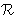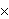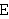Intuitionistic Logic Explorer < Previous   Next > Nearby theorems Mirrors  >  Home  >  ILE Home  >  Th. List  >  dfcnqs Unicode version

Theorem dfcnqs 7378
 Description: Technical trick to permit reuse of previous lemmas to prove arithmetic operation laws infrom those in. The trick involves qsid 6357, which shows that the coset of the converse epsilon relation (which is not an equivalence relation) acts as an identity divisor for the quotient set operation. This lets us "pretend" thatis a quotient set, even though it is not (compare df-c 7356), and allows us to reuse some of the equivalence class lemmas we developed for the transition from positive reals to signed reals, etc. (Contributed by NM, 13-Aug-1995.)
Assertion
Ref Expression
dfcnqsProof of Theorem dfcnqs
StepHypRef Expression
1 df-c 7356 . 22 qsid 6357 . 231, 2eqtr4i 2111 1Colors of variables: wff set class Syntax hints:wceq 1289cep 4114cxp 4436ccnv 4437cqs 6291cnr 6856cc 7348 This theorem was proved from axioms:  ax-1 5  ax-2 6  ax-mp 7  ax-ia1 104  ax-ia2 105  ax-ia3 106  ax-io 665  ax-5 1381  ax-7 1382  ax-gen 1383  ax-ie1 1427  ax-ie2 1428  ax-8 1440  ax-10 1441  ax-11 1442  ax-i12 1443  ax-bndl 1444  ax-4 1445  ax-14 1450  ax-17 1464  ax-i9 1468  ax-ial 1472  ax-i5r 1473  ax-ext 2070  ax-sep 3957  ax-pow 4009  ax-pr 4036 This theorem depends on definitions:  df-bi 115  df-3an 926  df-tru 1292  df-nf 1395  df-sb 1693  df-eu 1951  df-mo 1952  df-clab 2075  df-cleq 2081  df-clel 2084  df-nfc 2217  df-ral 2364  df-rex 2365  df-v 2621  df-sbc 2841  df-un 3003  df-in 3005  df-ss 3012  df-pw 3431  df-sn 3452  df-pr 3453  df-op 3455  df-br 3846  df-opab 3900  df-eprel 4116  df-xp 4444  df-cnv 4446  df-dm 4448  df-rn 4449  df-res 4450  df-ima 4451  df-ec 6294  df-qs 6298  df-c 7356 This theorem is referenced by:  axmulcom  7406  axaddass  7407  axmulass  7408  axdistr  7409
 Copyright terms: Public domain W3C validator